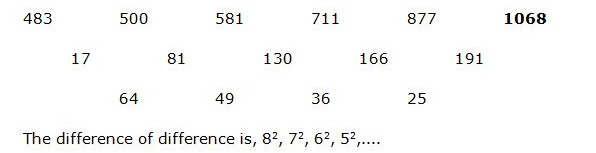# RRB PO Prelims Quantitative Aptitude (Day-01)

Dear Aspirants, Our IBPS Guide team is providing new series of Quantitative Aptitude Questions for RRB PO 2020 Prelims so the aspirants can practice it on a daily basis. These questions are framed by our skilled experts after understanding your needs thoroughly. Aspirants can practice these new series questions daily to familiarize with the exact exam pattern and make your preparation effective.

Start Quiz

1) The ratio of number of male and female in a committee is 8: 5. If the percentage of male and female increased by 25 % and 20 % respectively, then find the new ratio of male and female?

A.6: 5

B.3: 1

C.5: 3

D.7: 4

E.None of these

2) P, Q and R started a business by investing Rs. 25000, Rs. 30000 and Rs. 40000 respectively. After 5 months, P invested 25 % more But R withdraw Rs. 10000. Find the share of Q, if the total profit at the end of the year is Rs. 178200?

A.Rs. 68500

B.Rs. 57600

C.Rs. 72400

D.Rs. 63800

E.None of these

3) The base of the triangle is equal to the diagonal of the square, whose area is 288 Sq cm. Find the area of triangle, whose height is 32 cm?

A.384 Sq cm

B.456 Sq cm

C.420 Sq cm

D.472 Sq cm

1. E. None of these

4) If two dice are thrown simultaneously, then find the probability of getting a sum of dice is divisible by 5?

A.11/47

B.19/62

C.29/51

D.7/36

E.None of these

5) A Boat takes 4.5 hours less to travel to 144 Km downstream than to travel the same distance upstream. If the speed of the stream is 8 Km/hr, then find the speed of boat in still water?

A.16 km/hr

B.28 km/hr

C.24 km/hr

D.20 km/hr

E.None of these

Directions (6-10): What value should come in place of (?) in the following number series?

6) 483, 500, 581, 711, 877, ?

A.952

B.1124

C.996

D.1068

E.1156

7) 18, 21, 48, 153, 624, ?

A.2820

B.2675

C.3135

D.3350

E.3025

8) 117, 120, 125, 136, 161, ?

A.216

B.178

C.192

D.240

E.224

9) 17, 54, 165, 498, 1497,?

A.3246

B.2870

C.4288

D.4494

E.3852

10) 456, 228, 152, 114, ? , 76

A.98.4

B.91.2

C.106.6

D.103

E. 85

Given,

= > [8x * (125/100)] / [5x * (120/100)]

= > 5/3 = 5: 3

The share of P, Q and R

= [25000 * 5 + 25000 * (125/100) * 7]: [30000 * 12]: [40000* 5 + 30000* 7]

= > 343750: 360000: 410000

= > 275: 288: 328

891’s = 178200

1’s = 200

The share of Q = 288’s = Rs. 57600

The diagonal of the square = (1/2) * d2 = 288

d2 = 576

Diagonal of the square (d) = 24 cm

The area of triangle = (1/2) * b * h = (1/2) * 24 * 32 = 384 Sq cm

Total possible = 62 = 36

Possibilities of getting a sum of dice is divisible by 5

= > (1, 4), (2, 3), (3, 2), (4, 1), (4, 6), (5, 5), (6, 4) = 7

Required probability = 7/36

Let the speed of boat in still water be x km/hr,

According to the question,

[144/(x – 8)] – [144/(x + 8)] = (9/2)

[(x + 8) – (x – 8)]/[x2 – 64] = (9/2) * (1/144)

(16/[x2 – 64]) = 1/32

x2 – 64 = 512

x2 = 576

x = 24

The speed of boat in still water = 24 km/hr

Directions (6-10):18 * 1 + 3 = 21

21 * 2 + 6 = 48

48 * 3 + 9 = 153

153 * 4 + 12 = 624

624 * 5 + 15 = 3135

117 + (22 – 1) = 120

120 + (23 – 3) = 125

125 + (24 – 5) = 136

136 + (25 – 7) = 161

161 + (26 – 9) = 216

17 * 3 + 3 = 54

54 * 3 + 3 = 165

165 * 3 + 3 =498

498 * 3 + 3 = 1497

1497 * 3 + 3 = 4494

456 * ½ =228

228 * 2/3 = 152

152 * ¾ = 114

114 * 4/5 = 91.2

91.2 * 5/6 = 76